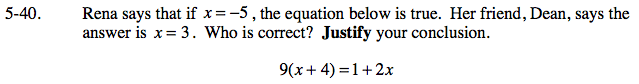Home > AC > Chapter 5 > Lesson 5.1.4 > Problem5-40

5-40.

Rena says that if x = −5, the equation below is true. Her friend, Dean, says the answer is x = 3. Who is correct? Justify your conclusion. Homework Help ✎

9(x + 4) = 1 + 2xSubstitute both numbers in for x and see which one makes the statement true.

If Joe said x = 2
9((2) + 4) = 1 + 2(2)
9(6) = 4
54 = 4

This statement is not true.

Now you try with the numbers given in the problem.